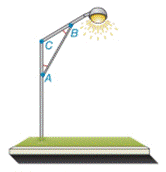Chapter 2.4, Problem 39E### Elementary Geometry for College St...

6th Edition
Daniel C. Alexander + 1 other
ISBN: 9781285195698

#### Solutions

Chapter
Section### Elementary Geometry for College St...

6th Edition
Daniel C. Alexander + 1 other
ISBN: 9781285195698
Textbook Problem
5 views

# A lamppost has design such that m ∠ C = 110 ° and ∠ A ≅ ∠ B . Find m ∠ A and m ∠ B .Exercises 39 ,   40

To determine

To find:

The value of mA and mB.

Explanation

Given:

mC=110° and AB

Figure (1)

Approach:

The sum of interior angles of a triangle is equal to 180°.

Calculation:

mA+mB+mC=180°

Substitute 110° for mC, and mA for mB in equation (1).

### Still sussing out bartleby?

Check out a sample textbook solution.

See a sample solution

#### The Solution to Your Study Problems

Bartleby provides explanations to thousands of textbook problems written by our experts, many with advanced degrees!

Get Started

#### 13. Write the equation of the line tangent to

Mathematical Applications for the Management, Life, and Social Sciences

#### Evaluate the definite integral. 04x1+2xdx

Single Variable Calculus: Early Transcendentals

#### Which is the best graph of y = f (x) for the given graph of y = f (x)? a) None of these

Study Guide for Stewart's Single Variable Calculus: Early Transcendentals, 8th